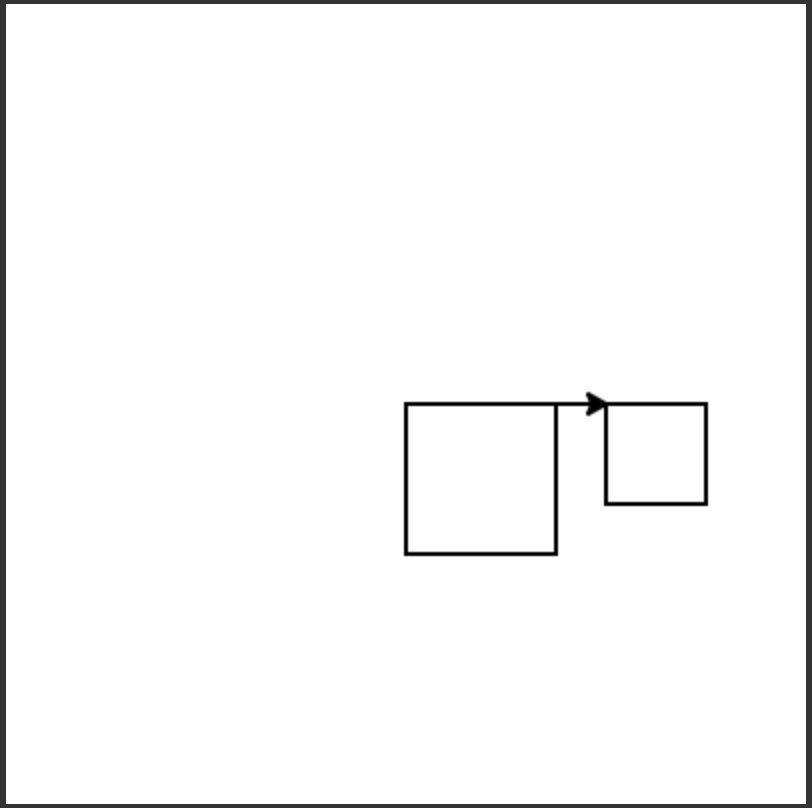# 6.4. Naming Input¶

What if we want to draw a different size square, like one with a side length of 50? We could change each of the calls to the `forward` procedure as shown below.

But, this means we have to change each of the four `forward` statements and we could make a mistake and not set all of them to the same number. Is there a better way? What if we create a variable `size` and set its value to the amount to move forward?

Now the program is easier to change since we only have one line to change `size = 50` to draw another size square. But, we still have to change the program in order to draw a different size square. Is there a better way?

We can add an additional input to the function that specifies the size of the square. Just separate the names for the inputs with a comma: `(turtle,size)` as shown below and be sure to specify the actual size when you call the procedure `square(malik, 100)` or `square(malik, 50)`.

The inputs that are specified in a function or procedure definition are also called parameters or formal parameters. So `turtle` and `size` are both parameters (formal parameters) in the `square` procedure. Notice that when we call `square` we have to specify the actual values for the inputs. The actual values passed into the function as inputs are called the arguments or actual parameters. In the call `square(malik, 50)` both `malik` and `50` are arguments (actual parameters) to the `square` procedure.

The following code assumes that a procedure square has been defined that takes a size. The code should create a turtle and then use it to draw a square, move forward, and draw a second square as shown below, but the lines are mixed up.Note

Discuss topics in this section with classmates.